# Regular event

(diff) ← Older revision | Latest revision (diff) | Newer revision → (diff)
A set of words over a finite alphabet which can be defined by using expressions of a special form, called regular expressions. Letbe a finite alphabet and let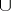,,be operation symbols, called union, concatenation and iteration, respectively. Regular expressions in the alphabetare defined inductively: 1) every letter ofis a regular expression; and 2) if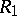,andare regular expressions, then so are,and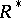. The language of regular expressions is interpreted in the following way.
Let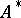be the set of all words over the alphabet, and let,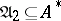. The symbol of any letter ofis regarded as a set consisting of one letter, andas the ordinary set-theoretical union. The set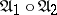consists of all words which are representable in the form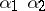where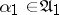,; if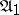andcontain the empty word, then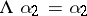,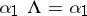and. Let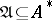, and for any, set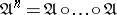(times). Thencoincides with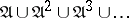; that is,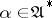if and only if there is an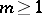such thatis representable in the form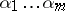, wherefor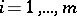. Thus a set of words over a finite alphabet is a regular event if and only if it can be obtained from one-letter sets using the operations of union, concatenation and iteration a finite number of times. Regular events can also be defined using other operations that preserve regularity (for example, intersection, taking complements, etc.), and also by presenting the set of words derivable in a formal system of the type of a semi-Thue system (see Thue system), by means of grammars, etc.
A set of subsets of words over a finite alphabet(of events) together with the operations introduced on this set form a certain algebra of events. The most important of these are algebras of regular events with operations that allow all regular events to be obtained from one-letter sets. Of the greatest interest is the question of finite axiomatizability of an algebra of regular events, that is, the question of the existence in such an algebra of a finite complete system of identities. In the most general formulation, the answer to this question is negative, although there are important subalgebras of algebras of regular events in which finite complete sets of identities exist.